×

Search anything:

# Beginner's Guide to Generative Adversarial Networks with a demo

#### Machine Learning (ML) GANsFREE BOOK -> Problems for the day before your Coding Interview (on Amazon)

Reading time: 40 minutes | Coding time: 20 minutes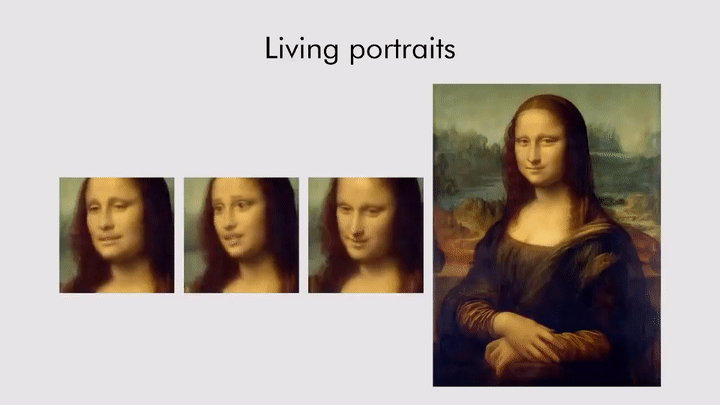Generative Adversarial Networks bring Mona Lisa to life!

Results from portrait of Mona Lisa via paper by Egor Zakharov et al.

I didn't want to start with a boring introduction for GANs, and so I straight away provided a proof of how cool they are!

Generative Adversarial Networks is the most interesting idea in the last 10 years in Machine Learning. — Yann LeCun, Director of AI Research at Facebook AI

Let's start demystifying them!

This post is broken into 5 parts:

• What are Generative adversarial networks(GANs)?
• Their architecture and working
• Mathematical background behind GANs
• Some interesting applications
• Code sample

## What is a GAN?

Generative Adversarial Network is an architecture for training a generative model. It was developed and introduced by Ian J. Goodfellow in 2014.

The architecture is comprised of two models:

• generator
• discriminator

The generator that we are interested in, and a discriminator model that is used to assist in the training of the generator. Initially, both of the generator and discriminator models were implemented as Multilayer Perceptrons (MLP) but recently, the models are implemented as deep convolutional neural networks.

GANs are neural networks that learn to create duplicate data similar to a known input data. The basic idea behind GANs is actually simple. GAN has two components with competing objectives that work through opposite goals. The generator tries to produce perfect data and the discriminator tries to find the defection in the data generated by the generator.

This results in generator improving its accuracy. This kind of situation can be modeled in Game Theory as a minimax game.

## GAN Architecture

Generative adversarial networks consist of two models: a generative model and a discriminative model.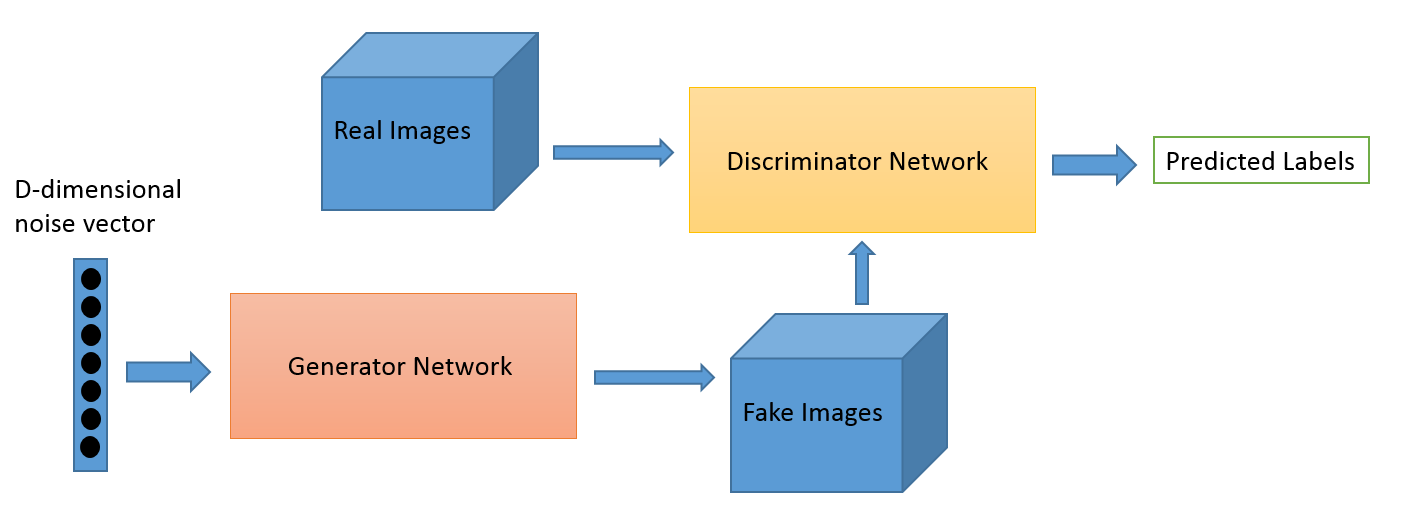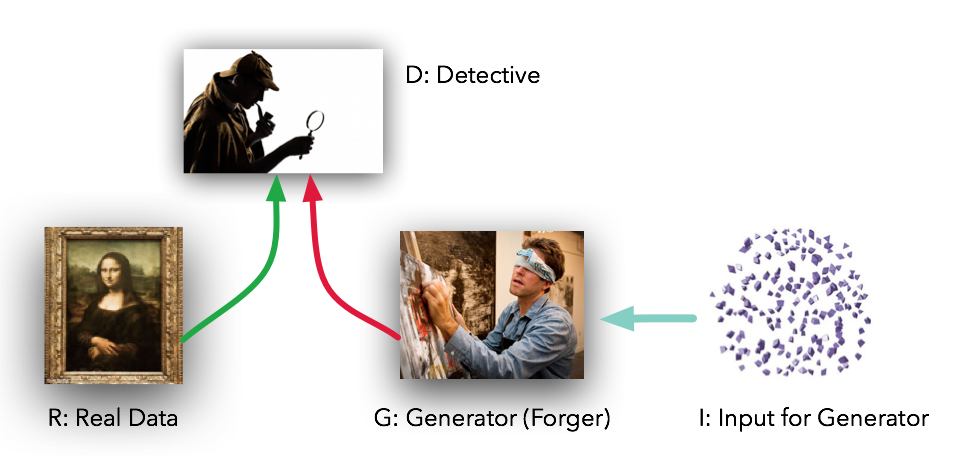The discriminator model is a classifier that has the task of determining whether a given image looks natural (an image from the dataset) or looks like it has been artificially created. This is basically a binary classifier that will take the form of a normal convolutional neural network (CNN).

The generator model takes random input values and transforms them into images through a deep convolutional neural network.

The generator is trying to fool the discriminator while the discriminator is trying to not get fooled by the generator. As the models are trained, both methods are improved until a point where the artificially created images are indistinguishable from the real ones. This is our goal.

## How does GAN work?

As we have seen above, the generator and discriminator are both neural networks, running in competition with each other in training phase.
The steps are repeated several times and in this, the Generator and Discriminator get better and better in their respective jobs after each repetition.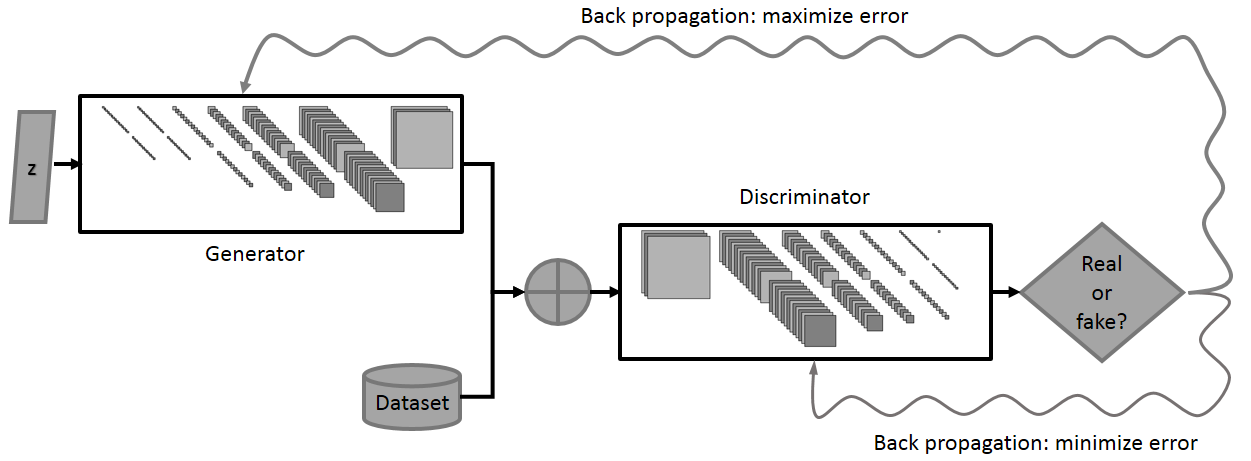There are really only 4 components to think about:

• R: The original, genuine data set
• I: The random noise that goes into the generator as a source of entropy
• G: The generator which tries to copy/mimic the original data set
• D: The discriminator which tries to tell apart G’s output from R

There are essentially two parts of training a GAN:

Part 1: Training discriminator

• The Discriminator is trained while the Generator is idle. In this phase, the network is only forward propagated and no back-propagation is done.
• The Discriminator is trained on both the real data and the fake generated data from Generator for n epochs, and see if it can correctly predict them as real and fake respectively.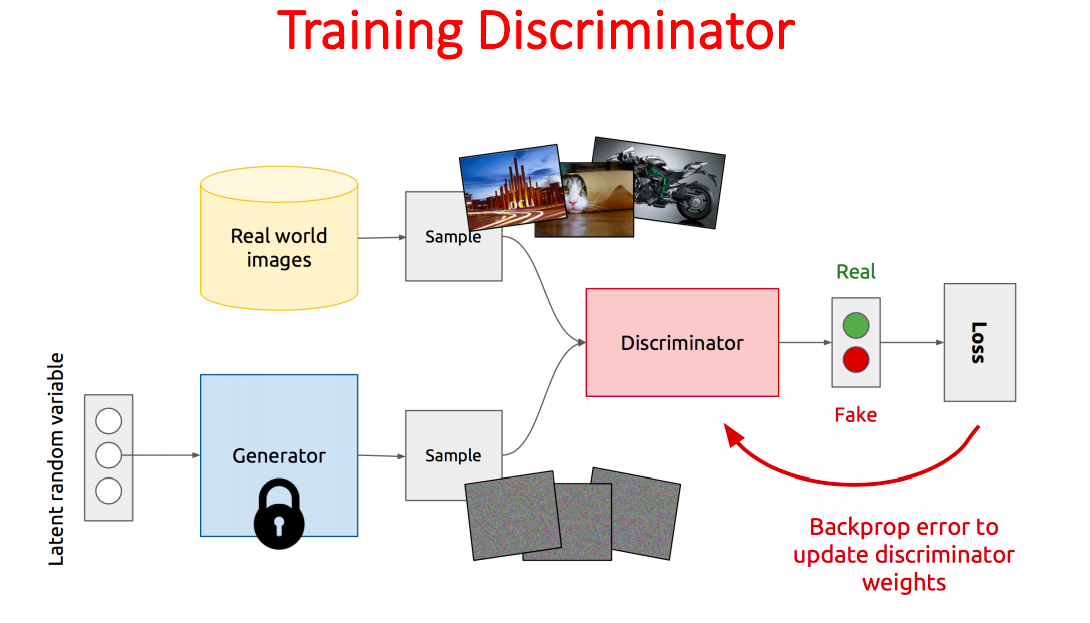Part 2: Training Generator

• The Generator is trained while the Discriminator is idle.
• After the Discriminator is trained by the generated fake data of the Generator, we can use its predictions to update the weights for Generator and make it smarter at fooling the Discriminator.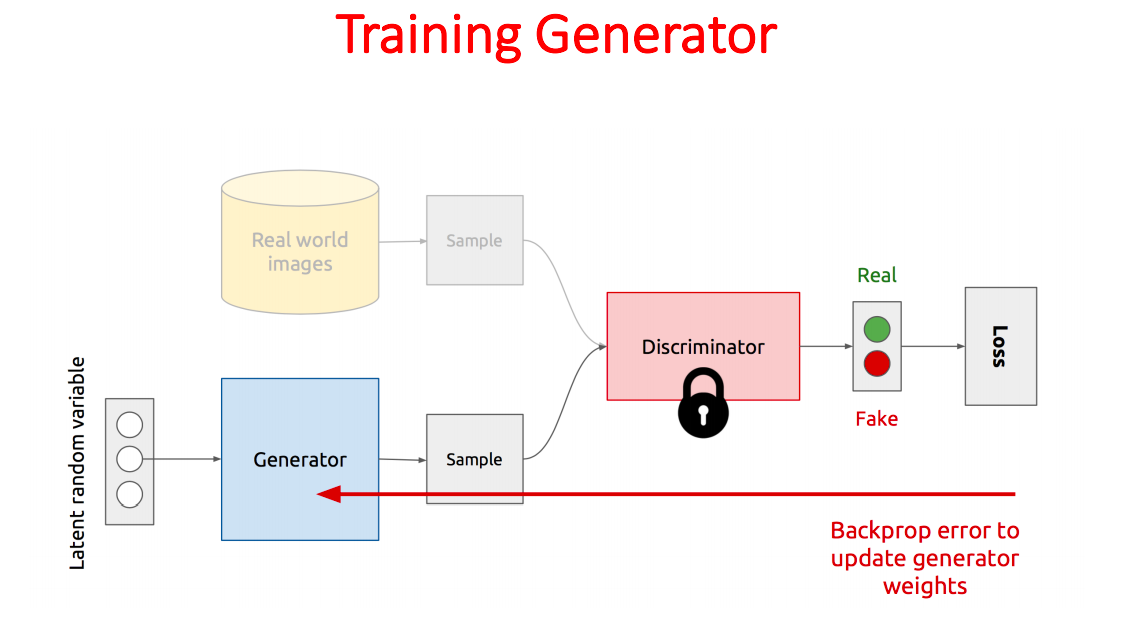## Mathematical intuition behind GANs

We are basically training the Discriminator to maximize the probability of assigning correct labels to both real and generated data.
We are also training the Generator to minimize the probability to get caught by the Discriminator, which is equivalent to minimizing log(1-D(G(z))).
The intuition behind this adversarial training is to reach the Nash equilibrium of a game, and therefore GANs are formulated a a minimax game where the Discriminator is trying to minimize its reward V(D, G) and the Generator is trying to minimize the Discriminator’s reward or in other words, maximize its loss.
Mathematically, we have: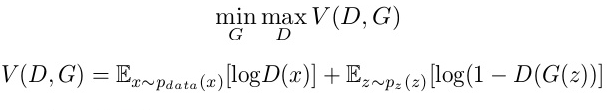where,
G = Generator
D = Discriminator
Pdata(x) = distribution of real data
P(z) = distribution of generator
x = sample from Pdata(x)
z = sample from P(z)
D(x) = Discriminator network
G(z) = Generator network

## Applications

Here are a few cool things possible with GANs

1. Generate Examples for Image Datasets/Data Augmentation: Aiming to reduce the need for labeled data (GAN is only used as a tool for enhancing the training process of another model).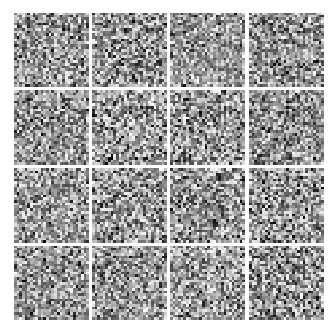Fashion MNIST GIF at different epochs

1. Face Aging: The process of generating photographs of faces with different apparent ages, from younger to older, using GANs

Research Paper: titled “Face Aging With Conditional Generative Adversarial Networks”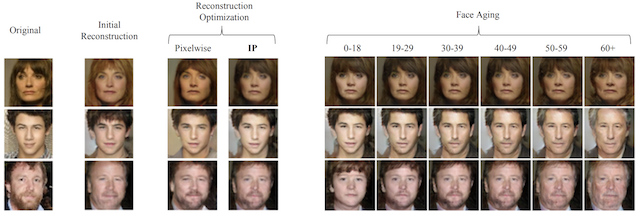1. Generate new human poses: Liqian Ma, et al. in their 2017 paper titled “Pose Guided Person Image Generation” provide an example of generating new photographs of human models with new poses.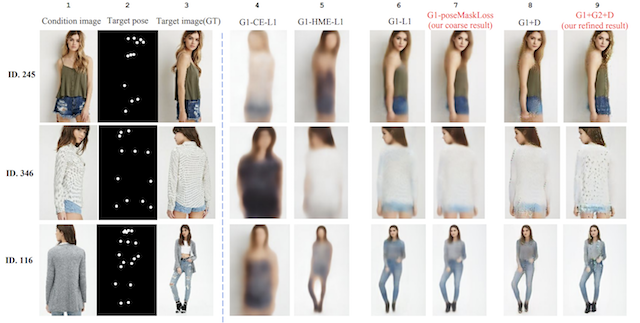1. Text-to-Image Translation (text2image): Han Zhang, et al. in their 2016 paper titled “StackGAN: Text to Photo-realistic Image Synthesis with Stacked Generative Adversarial Networks” demonstrate the use of GANs, specifically their StackGAN to generate realistic looking photographs from textual descriptions of simple objects like birds and flowers.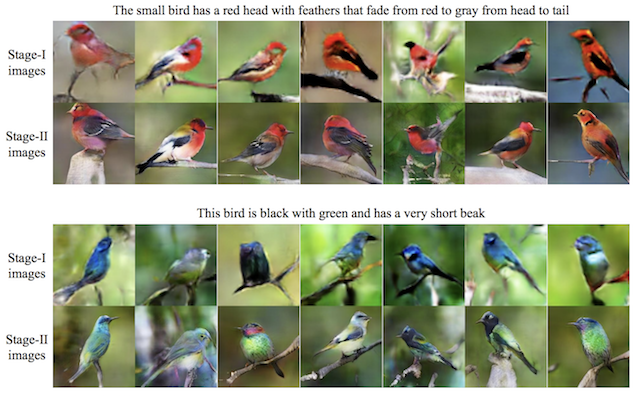1. Image-to-Image Translation:
Examples include translation tasks such as:
• Translation of satellite photographs to Google Maps.
• Translation of photos from day to night.
• Translation of sketches to color photographs.

Research Paper titled “Image-to-Image Translation with Conditional Adversarial Networks”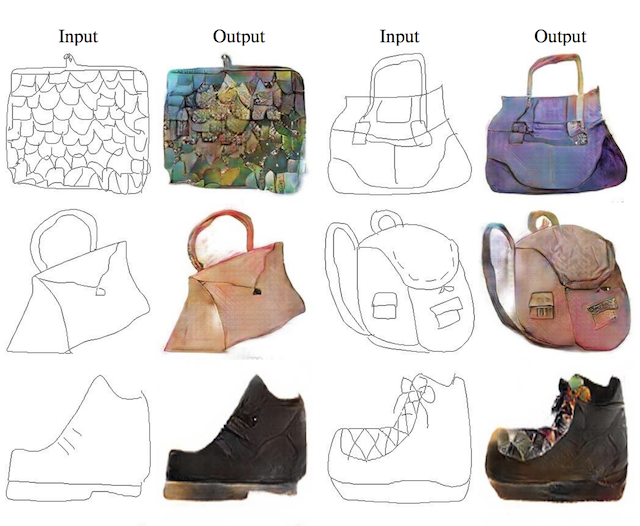## Let's get a hands on in GANs

We will generate the images from the famous Fashion MNIST dataset

1. Importing libraries
``````import os
import numpy as np
import matplotlib.pyplot as plt
from tqdm import tqdm

from keras.layers import Input
from keras.models import Model, Sequential
from keras.layers.core import Dense, Dropout
from keras.datasets import fashion_mnist
from keras import initializers
``````

Let's set values for some variables

``````# For consistent results
np.random.seed(10)

# The dimension of our random noise vector.
random_dim = 100
``````
``````def load_data():
(x_train, y_train), (x_test, y_test) = fashion_mnist.load_data()
# normalize our inputs to be in the range[-1, 1]
x_train = (x_train.astype(np.float32) - 127.5)/127.5
x_train = x_train.reshape(60000, 784)
return (x_train, y_train, x_test, y_test)
``````
1. Define model
We will be writing different functions for the generator and discriminator, so that they can be called separately.
They will then be combined to form a GAN network, where we will train them according to their logic.
``````def get_optimizer():
def get_generator(optimizer):
generator = Sequential()

generator.compile(loss='binary_crossentropy', optimizer=optimizer)
return generator

def get_discriminator(optimizer):
discriminator = Sequential()

discriminator.compile(loss='binary_crossentropy', optimizer=optimizer)
return discriminator

def get_gan_network(discriminator, random_dim, generator, optimizer):
# We initially set trainable to False since we only want to train # either the generator or discriminator at a time
discriminator.trainable = False
# gan input (noise) will be 100-dimensional vectors
gan_input = Input(shape=(random_dim,))
# the output of the generator (an image)
x = generator(gan_input)
# get the output of the discriminator (probability if the image # is real or not)
gan_output = discriminator(x)
gan = Model(inputs=gan_input, outputs=gan_output)
gan.compile(loss='binary_crossentropy', optimizer=optimizer)
return gan
``````
1. Plot generated images
``````def plot_generated_images(epoch, generator, examples=100, dim=(10, 10), figsize=(10, 10)):
noise = np.random.normal(0, 1, size=[examples, random_dim])
generated_images = generator.predict(noise)
generated_images = generated_images.reshape(examples, 28, 28)

plt.figure(figsize=figsize)
for i in range(generated_images.shape):
plt.subplot(dim, dim, i+1)
plt.imshow(generated_images[i], interpolation='nearest', cmap='gray_r')
plt.axis('off')
plt.tight_layout()
plt.savefig('gan_generated_image_epoch_%d.png' % epoch)
``````
1. Training time!
``````def train(epochs=1, batch_size=128):
# Get the training and testing data
x_train, y_train, x_test, y_test = load_data()
# Split the training data into batches of size 128
batch_count = x_train.shape / batch_size

# Build our GAN netowrk
gan = get_gan_network(discriminator, random_dim, generator, adam)

for e in range(1, epochs+1):
print ('-'*15, 'Epoch %d' % e, '-'*15)
for _ in tqdm(range(int(batch_count))):
# Get a random set of input noise and images
noise = np.random.normal(0, 1, size=[batch_size, random_dim])
image_batch = x_train[np.random.randint(0, x_train.shape, size=batch_size)]

# Generate fake Fashion MNIST images
generated_images = generator.predict(noise)
X = np.concatenate([image_batch, generated_images])

# Labels for generated and real data
y_dis = np.zeros(2*batch_size)
# One-sided label smoothing
y_dis[:batch_size] = 0.9

# Train discriminator
discriminator.trainable = True
discriminator.train_on_batch(X, y_dis)

# Train generator
noise = np.random.normal(0, 1, size=[batch_size, random_dim])
y_gen = np.ones(batch_size)
discriminator.trainable = False
gan.train_on_batch(noise, y_gen)

if e == 1 or e % 20 == 0:
plot_generated_images(e, generator)

if __name__ == '__main__':
train(400, 128)
``````
1. Results
The following images were generated after the first 20 epochs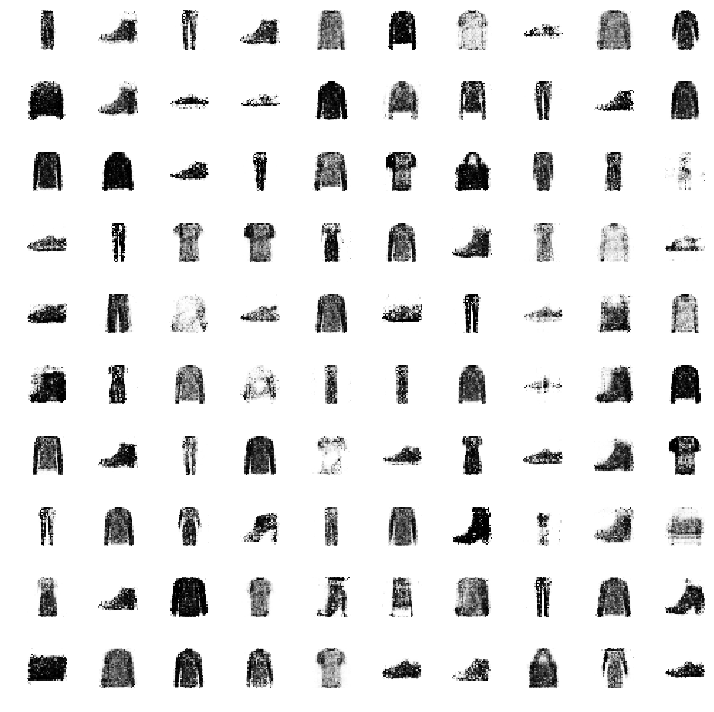In case, you want to look at the performance of this network on MNIST digits dataset, read this post where it was originally used.

By this, we come to the end of this post. If you are looking for a detailed description on how to code up GAN for an application such as Face Aging, head over to this post.